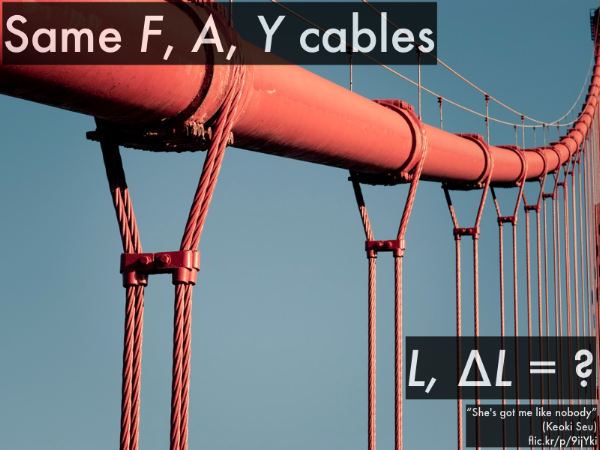## 20151104

Physics 205A, fall semester 2015
Cuesta College, San Luis Obispo, CA

Students have a bi-weekly online reading assignment (hosted by SurveyMonkey.com), where they answer questions based on reading their textbook, material covered in previous lectures, opinion questions, and/or asking (anonymous) questions or making (anonymous) comments. Full credit is given for completing the online reading assignment before next week's lecture, regardless if whether their answers are correct/incorrect. Selected results/questions/comments are addressed by the instructor at the start of the following lecture.

The following questions were asked on reading textbook chapters and previewing a presentation on elasticity.Selected/edited responses are given below.

Describe what you understand from the assigned textbook reading or presentation preview. Your description (2-3 sentences) should specifically demonstrate your level of understanding.
"Stress is applying a force to stretch something while strain is how the object responds to the stress."

"Tensile stress is applying a force to a certain cross section sample to stretch it. A compressive stress is an applying force to a certain cross section sample to squish it. Both tensile strain and compressive strain, measures how the material responds."

"Tension is stretching stuff thinner, compression is squishing stuff. Tensile stress is applying a force to stretch stuff and compressive stress is a force squishing stuff. Hooke's law gives the relationship of this stress (F/A), causing a strain (∆L/L) of a given material (Y)."

"Compressive stress is force applied on a cross section. Compressive strain is how the cross section responds to to the stress/ force applied."

Describe what you found confusing from the assigned textbook reading or presentation preview. Your description (2-3 sentences) should specifically identify the concept(s) that you do not understand.
"I found Hooke's Law tough to apply to real-life examples."

"Everything from your blog made sense, but the textbook confused me more than helped me honestly. They're are a few conceptional questions that I have that we will probably go over in class."

"Using the elastic deformation equation with Young's modulus."

"I have a hard time understanding Hooks law and how it works, it may be because I don't have a handle for what it actually is."

"The manipulation of different equations can be challenging when I am trying to conceptualize the problem."

"I understand most of it"

"I found nothing confusing--I am a genius."

What is the SI (Système International) unit for stress?
"Pa."

"N/m2."

"It does not have a unit."

"Newtons."

Explain why strain is a unitless quantity.
"When dividing a quantity of a particular unit by another quantity of the same units, the units cancel out and only a numerical value (the quotient of the quantities) remains."

"It is a measure of deformation--change in length divided by initial length cancels out the units."

"The fractional length change cause the units for length to cancel out, thus giving strain a unitless quantity--it is a relative change in shape."

"I don't know...please explain in class."

What is the SI (Système International) unit for Young's modulus?
"Pa."

"N/m2."

"Young's modulus is the ratio of stress (which has units of pressure) to strain (which is dimensionless), and so Young's modulus has units of pressure. Its SI unit is therefore the pascal."The __________ lengths of vertical suspension bridge cable are stretched by a greater amount ∆L from their original lengths.
 shorter. *********  longer. *******************************  (There is a tie.) ****************  (Unsure/lost/guessing/help!) *****The __________ columns of 2×4s support the least amount of force.
 narrower (two 2×4s). **************************  wider (three 2×4s). ****************  (There is a tie.) ***************  (Unsure/lost/guessing/help!) **** 

Ask the instructor an anonymous question, or make a comment. Selected questions/comments may be discussed in class.
"Is the material going to get easier or harder at this point in the semester?" (I think the only difference in the material from now on is that it is very compartmentalized, and does not critically rely on previous chapters for full understanding. After the build-up in the motion-forces-Newton's laws chapter sequence, this should be a good thing.)

The homework problems for the book are orders of magnitude more difficult than the rest of the coursework, I NEVER know how to do them and it makes me sad."

"The A in Hooke's law stands for area, right?" (Uh, yes.)

"Why is the notation '∆L' used for both tension and compression? Confusing." (If you start with a given length L, then ∆L represents how the length changes from that original amount, whether it gets slightly longer (due to stretching), or slightly shorter (due to squishing).)

"I'm going to get my cartilage pierced." (So, your cartilage will have a certain amount of stress applied to it, which will respond with...OW.)